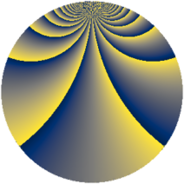# Properties

 Label 1152.3.uLevel $1152$ Weight $3$ Character orbit 1152.u Rep. character $\chi_{1152}(271,\cdot)$ Character field $\Q(\zeta_{8})$ Dimension $156$ Sturm bound $576$

# Learn more

## Defining parameters

 Level: $$N$$ $$=$$ $$1152 = 2^{7} \cdot 3^{2}$$ Weight: $$k$$ $$=$$ $$3$$ Character orbit: $$[\chi]$$ $$=$$ 1152.u (of order $$8$$ and degree $$4$$) Character conductor: $$\operatorname{cond}(\chi)$$ $$=$$ $$32$$ Character field: $$\Q(\zeta_{8})$$ Sturm bound: $$576$$

## Dimensions

The following table gives the dimensions of various subspaces of $$M_{3}(1152, [\chi])$$.

Total New Old
Modular forms 1600 164 1436
Cusp forms 1472 156 1316
Eisenstein series 128 8 120

## Trace form

 $$156q + 4q^{5} + 4q^{7} + O(q^{10})$$ $$156q + 4q^{5} + 4q^{7} - 4q^{11} - 4q^{13} + 4q^{19} + 60q^{23} - 4q^{25} + 4q^{29} - 100q^{35} - 4q^{37} + 4q^{41} - 92q^{43} - 8q^{47} - 156q^{53} + 260q^{55} - 132q^{59} + 60q^{61} + 8q^{65} + 36q^{67} + 252q^{71} - 4q^{73} + 228q^{77} - 504q^{79} - 484q^{83} + 96q^{85} + 4q^{89} - 188q^{91} - 8q^{97} + O(q^{100})$$

## Decomposition of $$S_{3}^{\mathrm{new}}(1152, [\chi])$$ into newform subspaces

The newforms in this space have not yet been added to the LMFDB.

## Decomposition of $$S_{3}^{\mathrm{old}}(1152, [\chi])$$ into lower level spaces

$$S_{3}^{\mathrm{old}}(1152, [\chi]) \cong$$ $$S_{3}^{\mathrm{new}}(32, [\chi])$$$$^{\oplus 9}$$$$\oplus$$$$S_{3}^{\mathrm{new}}(96, [\chi])$$$$^{\oplus 6}$$$$\oplus$$$$S_{3}^{\mathrm{new}}(128, [\chi])$$$$^{\oplus 3}$$$$\oplus$$$$S_{3}^{\mathrm{new}}(288, [\chi])$$$$^{\oplus 3}$$$$\oplus$$$$S_{3}^{\mathrm{new}}(384, [\chi])$$$$^{\oplus 2}$$